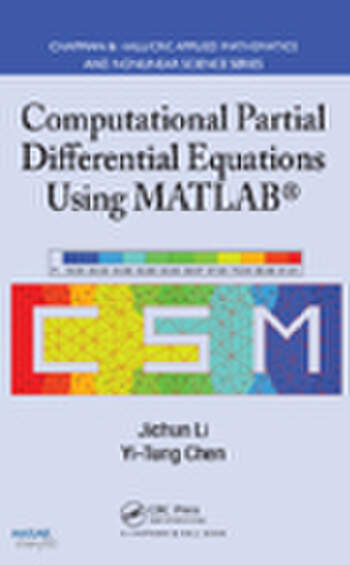# Computational Partial Differential Equations Using MATLAB

## 1st Edition

Jichun Li, Yi-Tung Chen

Chapman and Hall/CRC
Published October 20, 2008
Textbook - 378 Pages - 42 B/W Illustrations
ISBN 9781420089042 - CAT# C9048
Series: Textbooks in Mathematics

For Instructors Request Inspection Copy

was \$130.00

USD\$104.00

SAVE ~\$26.00

FREE Standard Shipping!

## Preview

### Summary

This textbook introduces several major numerical methods for solving various partial differential equations (PDEs) in science and engineering, including elliptic, parabolic, and hyperbolic equations. It covers traditional techniques that include the classic finite difference method and the finite element method as well as state-of-the-art numerical methods, such as the high-order compact difference method and the radial basis function meshless method.

Helps Students Better Understand Numerical Methods through Use of MATLAB®
The authors uniquely emphasize both theoretical numerical analysis and practical implementation of the algorithms in MATLAB, making the book useful for students in computational science and engineering. They provide students with simple, clear implementations instead of sophisticated usages of MATLAB functions.

All the Material Needed for a Numerical Analysis Course
Based on the authors’ own courses, the text only requires some knowledge of computer programming, advanced calculus, and difference equations. It includes practical examples, exercises, references, and problems, along with a solutions manual for qualifying instructors. Students can download MATLAB code from www.crcpress.com, enabling them to easily modify or improve the codes to solve their own problems.

#### Instructors

We provide complimentary e-inspection copies of primary textbooks to instructors considering our books for course adoption.